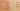ArraysGrow

Misconception:

One can add an arbitrary number of elements to an array, and the array will grow as needed.Incorrect

Arrays can grow dynamically

Correct

The length of an array is fixed and is determined at allocation

CorrectionHere is what's right.

The size of an array in Java is fixed and determined at the time it is allocated.

For example, when you write: new int, you get an array with space for exactly 19 elements of type int. The elements are set to their default value. The length of the array can be found by accessing its length field.

int[] a = new int;
a.length = 20; // ERROR

The value of the length field is constant, it cannot be changed.

int[] a = new int;
a = 10;
a = 11;
a = 12; // ERROR: ArrayIndexOutOfBoundsException

To “grow” an array, you need to allocate a new array, and copy the elements of your old array into the newly allocated longer array.

int[] a = new int;
a = 10;
a = 11;
int[] newA = new int[a.length + 1];
for (int i=0; i<a.length; i++) {
newA[i] = a[i];
}
a = newA;
a = 12; // OK, this new object has length 3

Alternatively you can use an ArrayList object, which takes care of allocating arrays to accommodate all its elements.

ArrayList<Integer> a = new ArrayList<Integer>();

OriginWhere could this misconception come from?

Students may be familiar with lists in Python (which grow with list.append(element)) or arrays in JavaScript (which grow with array.push(element)).

Java